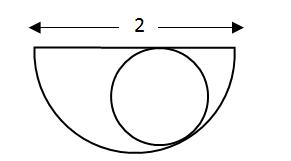# CAT Quantitative Aptitude Questions | Geometry Questions for CAT - Triangles

###### CAT Questions | CAT Geometry Questions | Circles

The question is from the topic circles. It discusses about a circle inside a semi-circle. CAT Geometry questions are heavily tested in CAT exam. Make sure you master Geometry problems. We have to find out the Radius of the circle inscribed inside a semi - circle

Question 37: A circle is inscribed in a semi-circle as shown:-The radius of the circle possibly is:-

1. (√2 + 1)/2
2. √2 − 1/2
3. 1 − √2
4. √2 − 1

## Best CAT Coaching in Chennai

#### CAT Coaching in Chennai - CAT 2021Online Batches Available Now!

##### Method of solving this CAT Question from Circles: Let's apply the details in formula directly.

Area of semicircle => (π * 12)/2 = 0.5 π
Area of circle should be less than the area of semicircle:-
Area = π * ((√2 + 1)/2)2 = π/4 * (2 + 1 + 2√2) = (0.75 + √2) * π > 0.5π
Area = π * (√2 − 1/2)2 = π * (2 + 1/4 − √2) = 0.85π > 0.5π
Area = π * (1 − 2√2)2 = π * (1 + 8 − 4√2) = (9 − 5.6)π = 3.4π > 0.5π
Area = π * (√2 − 1)2 =π * (2 + 1 − 2√2)=0.2π

The question is "Find the possible radius of the circle"

##### Hence, the answer is (√2 − 1).

Choice D is the correct answer.

###### CAT Coaching in ChennaiCAT 2021Enroll at 49,000/- 44,000/-

Online Classroom Batches Starting Now!

###### Best CAT Coaching in ChennaiPrices slashed by Rs 4000/-

Attend a Demo Class

## CAT Preparation Online | CAT Geometry questions Videos On YouTube

#### Other useful sources for Geometry Question | Geometry Triangles Circles Quadrilaterals Sample Questions

##### Where is 2IIM located?

2IIM Online CAT Coaching
A Fermat Education Initiative,
58/16, Indira Gandhi Street,
Kaveri Rangan Nagar, Saligramam, Chennai 600 093

##### How to reach 2IIM?

Phone: (91) 44 4505 8484
Mobile: (91) 99626 48484
WhatsApp: WhatsApp Now
Email: prep@2iim.com